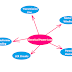In simple words, the unwanted consumption and dissipation of electrical power due to undesirable effects, energy conversion, and not use for any useful works can be called electrical power loss. Electricity generation is not free of cost so unwanted power loss can make a very effective economic loss. So we must aware of it and we should take action to prevent it as much as possible to reduce power loss.

Generally, we consider the electrical power loss when it is happen due to the current flow through a resistor and the electrical energy converted into heat energy.

Remember that when the electricity is used for the intention of heating then we can't call it electrical power loss.

Electrical power loss not only happens due to the conversion into heating energy. From a perspective of a healthy electrical system, there are a lot of reasons for the happening of electrical power loss.

## Causes of Electrical Power Loss

1. Electrical power loss happens due to the current flow through the unwanted resistance of a circuit.
2. Power Loss may happen due to a dead short circuit in a circuit that can cause an unwanted excessive current flow.
3. Power loss may happen due to the leakage of current flow in a circuit which also create unwanted excessive current flow in the circuit.
4. Electrical power loss may happen due to the low efficiency of the electrical equipment. When an equipment or device takes more electrical power than its output then we can say there is power loss happening.
5. Electrical power loss happens with some unwanted effects such as Parasitic Effect, Skinning Effect, Dielectric Loss, Corona Discharge, magnetic loss(hysteresis and eddy current), etc.

## Electrical Power Loss Examples

Some common examples of electrical power losses are,
• Power Loss in Transmission Line
• Iron Loss and Core Losses in Transformer Windings
• Iron Loss and Core Losses in Motor Winding
• Power losses in Capacitive and Inductive Loads
• Power losses in electronic circuits such as LCR circuits

Now, the question is how we know power loss happens in circuits. It is very simple if the output power is less than the input power of a system then there is some electrical power loss. The difference between output power and input power is the power loss.

Measuring electrical Power loss due to a resistive element is very easy. It is the simple multiplication of current flow and the value of resistance. Here is the formula,

P = I^2R

Here, P = Power Loss
I = Current flow through the resistor
R = Resistance Value

## Effects of Electrical Power Loss

1. Electrical Power loss in a resistive element can create unwanted heat in the circuit.
2. Power loss can reduce the efficiency of any electrical system or device.
3. Power loss can unbalance the electrical system.
4. Power loss can drop the receiving end voltage.

## How to Reduce Electrical Power Loss?

Before going to reduce the power loss we must know the actual cause of that power loss.
• If the power loss happens due to the unwanted resistance then we must try to reduce the resistance of the circuit or we can replace the resistive element with other elements. For example, the old electrical fan regulators work on the principle of current limiting using a resistor but there was a huge power loss. Nowadays, we use modern electronic fan regulators that work on the principle of chopping by semiconductor switches so there is very less power loss happening.
• In long-distance transmission lines, if the current flows more means the power also be more so to reduce the current flow the system voltage is increased by step-up transformers. And in the user end again it is stepped down by a step-down transformer.
• If the power loss happens due to the dielectric losses then use high constant and high-quality dielectric materials.
• Sometimes increasing of frequency can increase power losses so we have to maintain a certain frequency range to reduce the losses.
• Eddy current losses in transformer and motor winding cores are reduced by laminating the solid core. Instead of using a big solid core, multiple laminations can help to reduce the eddy current losses and excessive heating which will ultimately reduce the electrical power loss.

What is Electrical Power Loss? Its Causes, Examples, ReductionReviewed by Author on November 16, 2022 Rating: 5